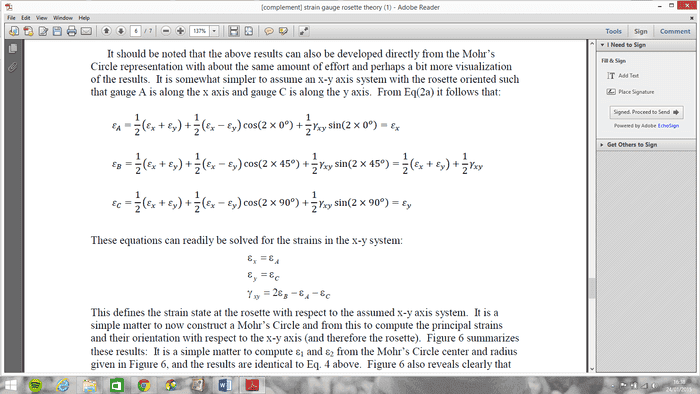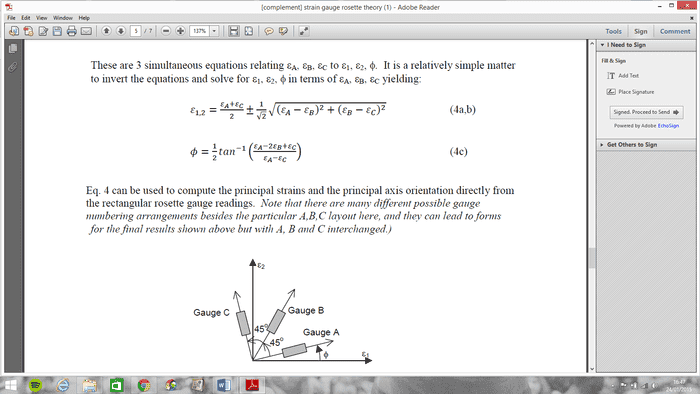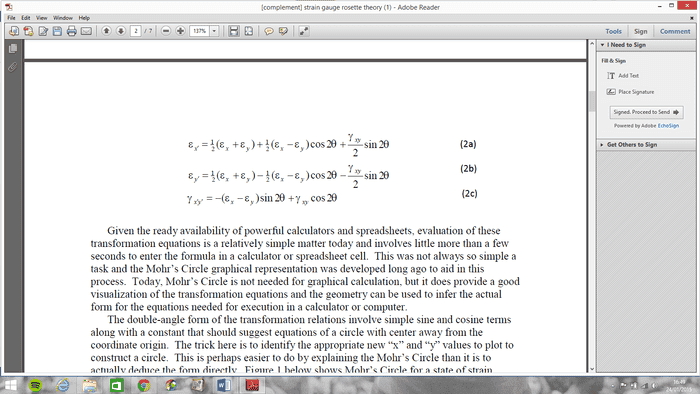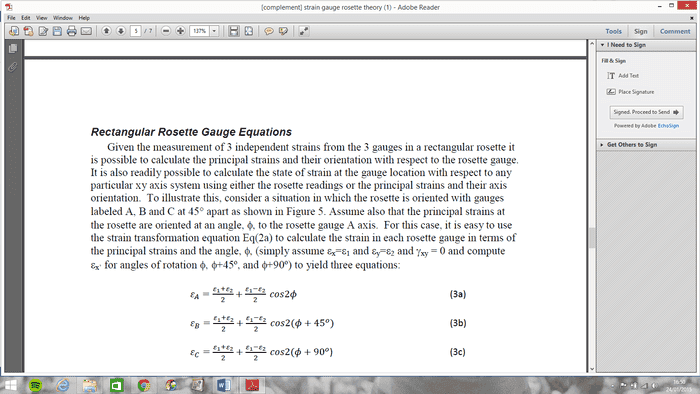# Mohr's Circle and Strain Gauges

Hi Guys,

I have been given a lab report to complete on Torsion of a beam.

- Beam was subjected to torsion under increments of weight
- Strain gauge rosette was placed, A B and C where there is 90 deg between A and C, B at 45 deg in between.
- The angle from Gauge A and the "x" axis was given as 28 deg

I have predicted a set of readings for A, B and C.

Now I have to construct a Mohr's circle from these values, relative to the shaft axis and find principal strains, principle direction and max sheer strain.

So far,

I used these to compute the strain readings to values for the mohrs circle.Then, I used these to find the Principal DirectionsBut then I am not sure how to use these equations for the ACTUAL orientation of the gauges/strains when I see these twoIf anybody has any ideas, I would be eternally grateful. I have been to tutors but they have been particularly unhelpful.

If anyone prefers to PM or Skype just let me know and I can explain my work so far.

You have strains ##\epsilon_A, \epsilon_B, \epsilon_C## and you plug them into the equations for ##\epsilon_1, \epsilon_2, \phi## given for rectangular rosettes (assuming you used rectangular rosettes, but if you used deltas then use the delta equations). The rest is just derivation to explain where those equations come from and how to relate them to Mohr's circle. The orientation is given by ##\phi## from the principal direction to the gage axis.

I converted the strains to εx, εy and γxy (2nd eq. 1st screenshot)

I used the εA, εB εC values from before to find the principal strains ε1 ε2 (eq 4a)

So I plotted this, and I work out the angle θ = -16.26 degrees.

However, Where does my value Φ come into it? My value is given as 28 degrees as the angle between gauge A and ε1?

You've got three angles you're working with:
1. 28 deg between the gage A axis and your chosen x-axis (we can call that Ψ)
2. -16.26 deg between the x-axis and the major principal direction (your angle θ, assuming it's the θ used in Mohr's circle and that you calculated it right)
3. The angle between the gage A axis and the major principal direction (the angle Φ that you will calculate using Eq. 4c). This can be calculated directly from the gage strains A, B, and C.

3. I have been given Φ as 28 degrees.

I have the orientation of the the strain gauges NOT relative to the 28 degrees

- The angle from Gauge A and the "x" axis was given as 28 deg

Didn't you state in the OP that the angle between gage A and the x-axis was 28 deg?

I've been given the angle between Gauge A and the axis of the Shaft (subjected to torsion) This is what the 28 degrees is

When I've drawn the circle, I get the angle of -16 degrees from the Principal direction and the x axis.

I dont understand how these two angles work together- do I add them?

I think the problem you're having is more conceptual, meaning that you're not quite understanding what the numbers mean that you're calculating. I'll try my best to answer the conceptual ones, but I don't want to hand you the "add 2 more to 3 to make 5" solution.

I hear you mate, no problems.

Thanks anyway